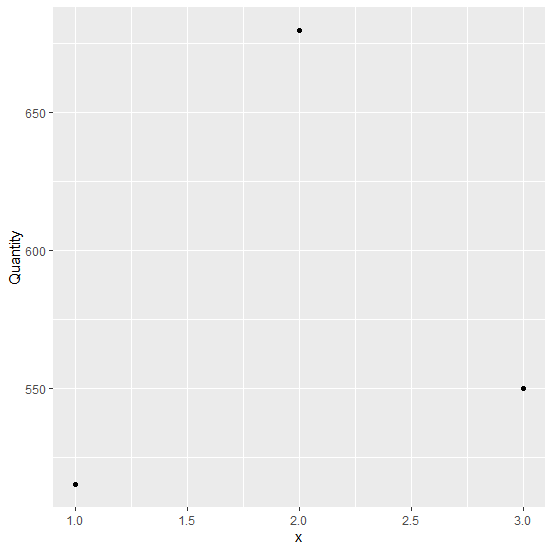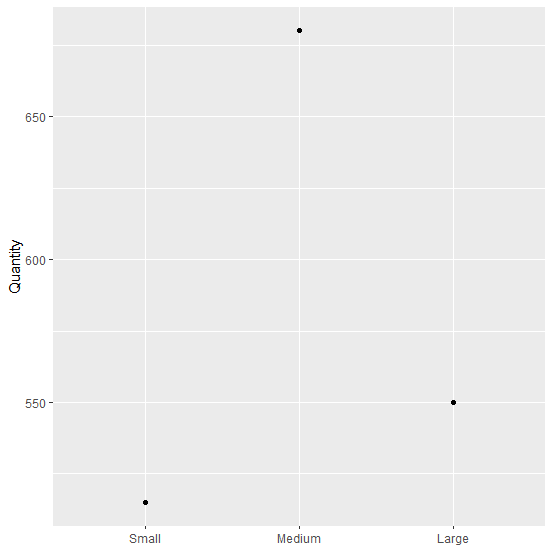# How to change ordinal X-axis label to text labels using ggplot2 in R?

A plot created with ordinal values on X-axis needs to be ordered for plotting, otherwise, the plot will have continuous values on the X-axis that includes ordinal values. If we want to convert those values to text then scale_x_discrete should be used with the number of breaks, these number of breaks are the actual number of labels we want to use in our plot.

## Example

Consider the below data frame −

x<-1:3
Quantity<-c(515,680,550)
df<-data.frame(x,Quantity)
library(ggplot2)
ggplot(df,aes(x,Quantity))+geom_point()

## OutputPlotting text labels on X-axis in place of numbers −

ggplot(df,aes(ordered(x),Quantity))+geom_point()+
+ scale_x_discrete(breaks = 1:3, labels=c("Small","Medium","Large"))+
+ xlab(NULL)

## Output